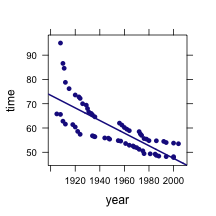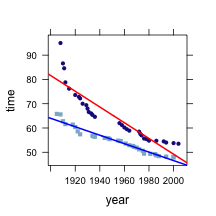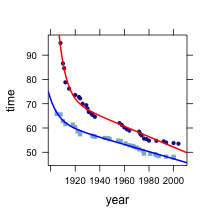# Linear Models of Relationships

``````library(mosaic, quietly = TRUE)
trellis.par.set(theme = col.mosaic())
``````

Rather than treating linear relationships abstractly, use them as models.

## Swimming World Records

These are world records in the 100m freestyle.

``````swim = fetchData("swim100m.csv")
``````
``````## Retrieving data from http://www.mosaic-web.org/go/datasets/swim100m.csv
``````
``````xyplot(time ~ year, data = swim)
mod = fitModel(time ~ A + B * year, data = swim)
plotFun(mod(year) ~ year, add = TRUE)
``````#### Questions:

1. What does the model tell you about how world records change?\
2. According to the model, what will be the world record in 2020? Answer: `42.2903`
3. According to the model, what was the hypothetical world record in year 0? Why is this not a realistic proposition?
4. What obvious features about the data does the model fail to capture?

### An elaboration

Men's and women's world records are different. Let's model them separately.

``````modF = fitModel(time ~ A + B * year, data = subset(swim, sex == "F"))
modM = fitModel(time ~ A + B * year, data = subset(swim, sex == "M"))
xyplot(time ~ year, group = sex, data = swim)
plotFun(modF(year) ~ year, add = TRUE, col = "red")
plotFun(modM(year) ~ year, add = TRUE, col = "blue")
``````#### QUESTIONS

1. How much faster are women's records improving than men's?
2. According to the model, when will the women's record be better than the men's?
3. What broad features are in the data are not represented by the model?

### Further elaboration

For a later example, model this as an exponential decay along with a linear improvement. What does this say about how the records are going to change into the future. The fit is numerically difficult. Reasonable initial guesses about the parameters need to be provided for the fitter to work.

``````modF2 = fitModel(time ~ A + B * year + C * exp(-k * (year - 1900)),
start = list(A = 40, B = -1, C = 30, k = log(2)/10), data = subset(swim,
sex == "F"))
modM2 = fitModel(time ~ A + B * year + C * exp(-k * (year - 1900)),
start = list(A = 40, B = -1, C = 30, k = log(2)/10), data = subset(swim,
sex == "M"))
xyplot(time ~ year, group = sex, data = swim)
plotFun(modF2(year) ~ year, add = TRUE, col = "red")
plotFun(modM2(year) ~ year, add = TRUE, col = "blue")
``````#### Questions:

1. According to the model, when will the women's record beat the men's?
2. Compare this model to an exponential model without the linear trend. Which model is preferred? Are your reasons related just to the match with the data or is there a more fundamental reason for your preference?

## Natural Gas Use versus Temperature

The data in `utilities.csv` are about the utility use in a home in St. Paul, Minnesota. What's the relationship between `thermsPerDay` and temperature `temp`?

## Wage versus Education

The data in `cps.csv` gives information about hourly wages in the 1970s. 1. What's the relationship between wage and education level?

2. Is this relationship evident by eye from a plot?
3. What other variables might influence wage? Try including them and seeing how the relationship with education level chages.
4. Does it make sense to talk about there being a particular relationship when it depends on what other variables are being considered?